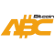Bitcoin ABC  0.27.6 P2P Digital Currency
BCLog Namespace Reference

## Classes

class  Timer
RAII-style object that outputs timing information to logs. More...

class  Logger

## Enumerations

enum  LogFlags : uint32_t {
NONE = 0 , NET = (1 << 0) , TOR = (1 << 1) , MEMPOOL = (1 << 2) ,
HTTP = (1 << 3) , BENCH = (1 << 4) , ZMQ = (1 << 5) , WALLETDB = (1 << 6) ,
RPC = (1 << 7) , ESTIMATEFEE = (1 << 8) , ADDRMAN = (1 << 9) , SELECTCOINS = (1 << 10) ,
REINDEX = (1 << 11) , CMPCTBLOCK = (1 << 12) , RAND = (1 << 13) , PRUNE = (1 << 14) ,
PROXY = (1 << 15) , MEMPOOLREJ = (1 << 16) , LIBEVENT = (1 << 17) , COINDB = (1 << 18) ,
QT = (1 << 19) , LEVELDB = (1 << 20) , VALIDATION = (1 << 21) , AVALANCHE = (1 << 22) ,
I2P = (1 << 23) , CHRONIK = (1 << 24) , BLOCKSTORE = (1 << 26) , ALL = ~uint32_t(0)
}

## Functions

std::string LogEscapeMessage (const std::string &str)
Belts and suspenders: make sure outgoing log messages don't contain potentially suspicious characters, such as terminal control codes. More...

## ◆ LogFlags

 enum BCLog::LogFlags : uint32_t
Enumerator
NONE
NET
TOR
MEMPOOL
HTTP
BENCH
ZMQ
WALLETDB
RPC
ESTIMATEFEE
SELECTCOINS
REINDEX
CMPCTBLOCK
RAND
PRUNE
PROXY
MEMPOOLREJ
LIBEVENT
COINDB
QT
LEVELDB
VALIDATION
AVALANCHE
I2P
CHRONIK
BLOCKSTORE
ALL

Definition at line 38 of file logging.h.

## ◆ LogEscapeMessage()

 std::string BCLog::LogEscapeMessage ( const std::string & str )

Belts and suspenders: make sure outgoing log messages don't contain potentially suspicious characters, such as terminal control codes.

This escapes control characters except newline ('
') in C syntax. It escapes instead of removes them to still allow for troubleshooting issues where they accidentally end up in strings.

Definition at line 217 of file logging.cpp.

Here is the caller graph for this function: